## Example Questions

← Previous 1 3 4 5 6 7 8 9 19 20

### Example Question #1 : How To Order Fractions From Least To Greatest Or From Greatest To Least

Order the following fractions from least to greatest:

2/3, 3/4, 1/2

3/4, 2/3, 1/2

2/3, 3/4, 1/2

3/4, 1/2, 2/3

1/2, 2/3, 3/4

2/3, 1/2, 3/4

1/2, 2/3, 3/4

Explanation:

1/2 is the least, then 2/3, then 3/4.

### Example Question #2 : How To Order Fractions From Least To Greatest Or From Greatest To Least

Place the following fractions in order from greatest to least.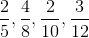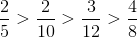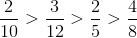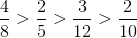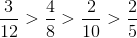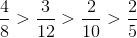Explanation:

This question can be most easily answered by converting the fractions into decimals first.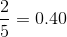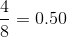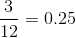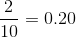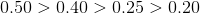### Example Question #3 : How To Order Fractions From Least To Greatest Or From Greatest To Least

Order the following fractions from least to greatest: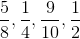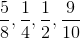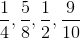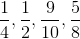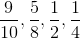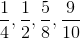Explanation:

The most generic process of ordering fractions that can apply to all questions begins with finding the common denominator between all fractions, preferably the least common denominator. In this question, the LCD is 40. Therefore the fractions become: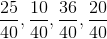. From this point, we can simply arrange the fractions based upon their numerators. This becomes: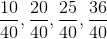. The final step is to reduce the fractions to their original denominators, which becomes:.

If you are comfortable and competent in quickly converting fractions to decimals, then there is a quicker method than what is described above. Simply convert each fraction to a decimal, organize the decimals, and then convert the decimals back to fractions. In this question we can convertto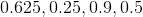. Then we can reorganize this to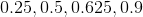and convert back to.

When approaching this kind of problem, choose the method in which you are more comfortable working with. There is no sense in trying to save time by choosing a method that you are not comfortable with and increasing your risk of answering the question incorrectly.

### Example Question #4 : How To Order Fractions From Least To Greatest Or From Greatest To Least

Place the following fractions in increasing order: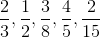.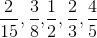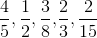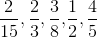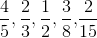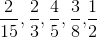Explanation:

To put these fractions in increasing order it is important to compare them as decimals, since fractions can often be deceiving.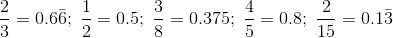.

Now that the value of each fraction is obvious we can sort then in increasing order:### Example Question #1 : Fractions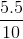is equivalent to what percentage?

5.5%

4.5%

15%

45%

55%

55%

Explanation:

Divide the fraction out to get the decimal

5.5 / 10 = 0.55

To find the percentage, shift the decimal point two to the right = 55%

### Example Question #2 : Fractions

Write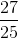as a percent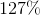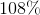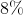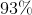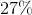Explanation:

To find a percent from a fraction you can either divide the number on your calculator and multiply by 100: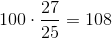or you can just multiply the numerator and denominator by the same number that makes the denominator equal to 100 (because a % is just #/100):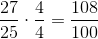### Example Question #1 : Fractions

One half of a group of professors is made up of mathematicians, and one fourth of the remaining portion is made up of philosophers. The remaining portion is made up of sociologists. If there are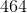professors in the room, how many are sociologists?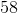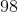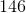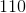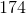Explanation:

To begin, remember with percentages that "of" means multiplication and "is" means "equals." Now, we know that one fourth of the remaining half are philosophers. This means that he philosophers really are: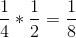So, there are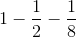sociologists as a percentage. This is: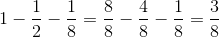Therefore,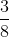of theare sociologists, or: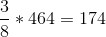are sociologists.

### Example Question #4 : Fractions

Convert the following fraction into a percent.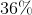Explanation:

To convert a fraction into a percent, make the denominator 100 and then the numerator will be the percent. Thus,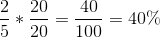### Example Question #5 : Fractions

On a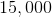acre plot of land, there areacres in which deer live. What percentage of the land does this represent? Round to the nearest hundredth.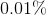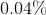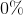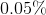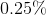Explanation:

For percentages, it is always easiest to translate into language that uses "is" and "of." "Is" means "equals" and "of" means "multiply." So, we have:

What percentage ofis? This is the same as: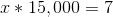Solving for, you get: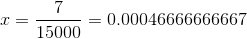Now, as a percent, this is equal to: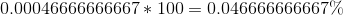Rounding to the nearest hundredth, you have.

### Example Question #6 : Fractionsis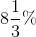of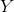, which is 35% of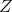. All three are positive integers. In which range does the smallest possible value of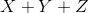fall?

Between 300 and 400

Between 500 and 600

Between 400 and 500

Between 600 and 700

Between 700 and 800

Between 300 and 400

Explanation:is 35% of, so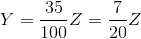isof, so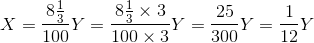.

Consequently,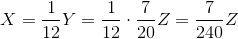The smallest integercan be is 240. If this happens,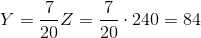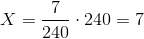Their sum is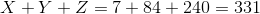The correct choice is that the sum is between 300 and 400.

← Previous 1 3 4 5 6 7 8 9 19 20

### All ACT Math Resources